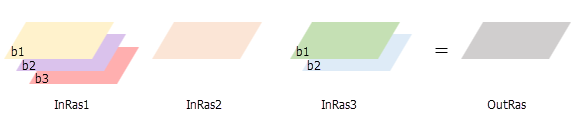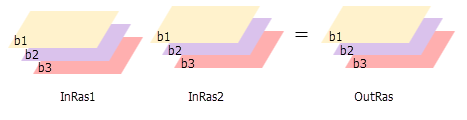# Cell Statistics (Image Analyst)

## Summary

Calculates a per-cell statistic from multiple rasters.

The available statistics are Majority, Maximum, Mean, Median, Minimum, Minority, Range, Standard deviation, Sum, and Variety.

## Usage

• The order of the input rasters is irrelevant for this tool.

• For the Maximum, Minimum, Mean, Median, Majority, Minority and Sum statistic types, if a single raster is used as the input, the output cell values will be the same as the input cell values. For Range and Standard deviation, the output cell values will all be 0. For Variety, it will be 1.

• If the Process as multiband parameter is unchecked (process_as_multiband is set to SINGLE_BAND in Python), each band from a multiband raster input will be processed separately as a single band raster, and the output will be a single band raster.• If the Process as multiband parameter is checked (process_as_multiband is set to MULTI_BAND in Python), each multiband raster input will be processed as a multiband raster, and the output will be a multiband raster. The output raster will also be multiband if the inputs are a combination of a multiband raster and constants. The number of bands in each multiband input must be the same.

The tool will perform the operation on each band from one input using the corresponding band from the other input. If one of the inputs is a multiband raster and the other input is a constant, the tool will perform the operation using the constant value for each band in the multiband input.## Syntax

`CellStatistics(in_rasters_or_constants, {statistics_type}, {ignore_nodata}, {process_as_multiband})`
 Parameter Explanation Data Type in_rasters_or_constants[in_raster_or_constant,...] A list of input rasters for which a statistic will be calculated for each cell in the Analysis window.A number can be used as an input; however, the cell size and extent must first be set in the environment.If the processing_as_multiband parameter is set to MULTI_BAND, all multiband inputs should have an equal number of bands. Raster Layer; Constant statistics_type(Optional) Specifies the statistic type to be calculated.MEAN —The mean (average) of the inputs will be calculated.MAJORITY —The majority (value that occurs most often) of the inputs will be calculated.MAXIMUM —The maximum (largest value) of the inputs will be calculated.MEDIAN —The median of the inputs will be calculated.MINIMUM —The minimum (smallest value) of the inputs will be calculated.MINORITY —The minority (value that occurs least often) of the inputs will be calculated.RANGE —The range (difference between largest and smallest value) of the inputs will be calculated.STD —The standard deviation of the inputs will be calculated.SUM —The sum (total of all values) of the inputs will be calculated.VARIETY —The variety (number of unique values) of the inputs will be calculated.The default statistic type is Mean. String ignore_nodata(Optional) Specifies whether NoData values will be ignored by the statistic calculation.DATA —At the processing cell location, if any of the input rasters has NoData, that NoData value will be ignored. The statistics will be computed by only considering the cells with valid data. This is the default.NODATA —If the processing cell location for any of the input rasters is NoData, the output for that cell will be NoData. Boolean process_as_multiband(Optional) Specifies how the input multiband raster bands will be processed. SINGLE_BAND —Each band from a multiband raster input will be processed separately as a single band raster. This is the default.MULTI_BAND —Each multiband raster input will be processed as a multiband raster. The operation will be performed for each band from one input using the corresponding band number from the other inputs. Boolean

#### Return Value

 Name Explanation Data Type out_raster The output raster.For each cell, the value is determined by applying the specified statistic type to the input rasters at that location. Raster

## Code sample

CellStatistics example 1 (Python window)

This example calculates the standard deviation per cell on several input Grid rasters and outputs the result as an IMG raster.

``````import arcpy
from arcpy import env
from arcpy.ia import *
env.workspace = "C:/iapyexamples/data"
outCellStats = CellStatistics(["degs", "negs", "cost"], "STD", "DATA")
outCellStats.save("C:/iapyexamples/output/outcellstats.img")``````
CellStatistics example 2 (stand-alone script)

This example calculates the range per cell per band on several input multiband rasters and creates a multiband output raster.

``````# Name: CellStatistics_Ex_standalone.py
# Description: Calculates a per-cell statistic from multiple multiband rasters
#               and process as multiband.
# Requirements: Image Analyst Extension

# Import system modules
import arcpy
from arcpy.ia import *

# Check out the ArcGIS Image Analyst extension license
arcpy.CheckOutExtension("ImageAnalyst")

# Set the analysis environments
arcpy.env.workspace = "C:/sapyexamples/data"

# Set the local variables
inRaster01 = "degs_MB"
inRaster02 = "negs_MB"
inRaster03 = "cost_MB"

# Execute CellStatistics
outCellStatistics = CellStatistics([inRaster01, inRaster02, inRaster03], "RANGE", "NODATA", "MULTI_BAND")

# Save the output
outCellStatistics.save("C:/sapyexamples/output/cellstats_MB.tif")``````

## Licensing information

• Basic: Requires Image Analyst or Spatial Analyst
• Standard: Requires Image Analyst or Spatial Analyst
• Advanced: Requires Image Analyst or Spatial Analyst